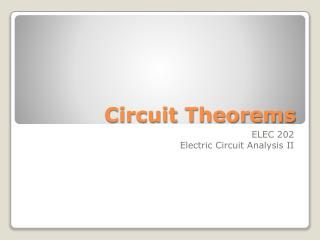DownloadDownload PresentationCircuit Theorems

# Circuit Theorems

Download Presentation## Circuit Theorems

- - - - - - - - - - - - - - - - - - - - - - - - - - - E N D - - - - - - - - - - - - - - - - - - - - - - - - - - -
##### Presentation Transcript

1. Circuit Theorems ELEC 202 Electric Circuit Analysis II

2. Superposition Principle The voltage across or current through an element in a linear circuit with multiple independent sources can be determined as the algebraic sum of such voltages or currents due to each source acting alone one at a time.

3. Observations The total response (voltage or current) is the sum of the responses contributed by each independent source separately. Superposition cannot be used for calculating POWER (not a linear quantity). Voltage source is turned off or deactivated by replacing it with a short circuit. Current source is turned off or deactivated by replacing it with an open circuit.

4. Observations Dependent sources are left intact. The response due to each active source can be determined by using basic circuit analysis laws and techniques (Ohm’s, KVL, KCL, voltage and current divider, and series/parallel combinations.)

5. Superposition for AC Circuits Usual procedures for DC circuits apply. However, phasor transformation must be carefully carried out if the circuit has sources operating at different frequencies  a different phasor circuit for each source frequency because impedance is a frequency-dependent quantity. inductor  a short circuit for DC capacitor  an open circuit for DC

6. Superposition for AC Circuits For sources of different frequencies, the total response must be added in the time domain DO NOT ADD INDIVIDUAL RESPONSES IN THE PHASOR DOMAIN IF THE SOURCES HAVE DIFFERENT FREQUENCIES.

7. Example 1 Find I0 in the circuit using superposition.

8. Example 1 (cont’d)

9. Example 1 (cont’d)

10. Example 2 Find v0 in the circuit using superposition.

11. Example 2 (cont’d)

12. Example 2 (cont’d)

13. Example 2 (cont’d)

14. Thevenin and NortonEquivalent Circuits Thevenin’s and Norton’s Theorems can be used to analyze AC circuits in the same way as in the analysis of DC circuits. 3 cases of interest: a) independent sources only, no dependent sources; b) both independent and dependent sources; c) dependent sources only, no independent sources;

15. Thevenin Equivalent Circuits

16. Norton Equivalent Circuits

17. Example 1 Obtain the Thevenin equivalent circuit at terminals a-b.

18. Example 1 (cont’d)

19. Example 1 (cont’d)

20. Example 2 Obtain the Thevenin equivalent circuit at terminals a-b.

21. Example 2 (cont’d)

22. Example 2 (cont’d)

23. Example 3 Obtain current Io using Norton’s theorem at terminals a-b.

24. Example 3 (cont’d)

25. Example 3 (cont’d)

26. Example 3 (cont’d)